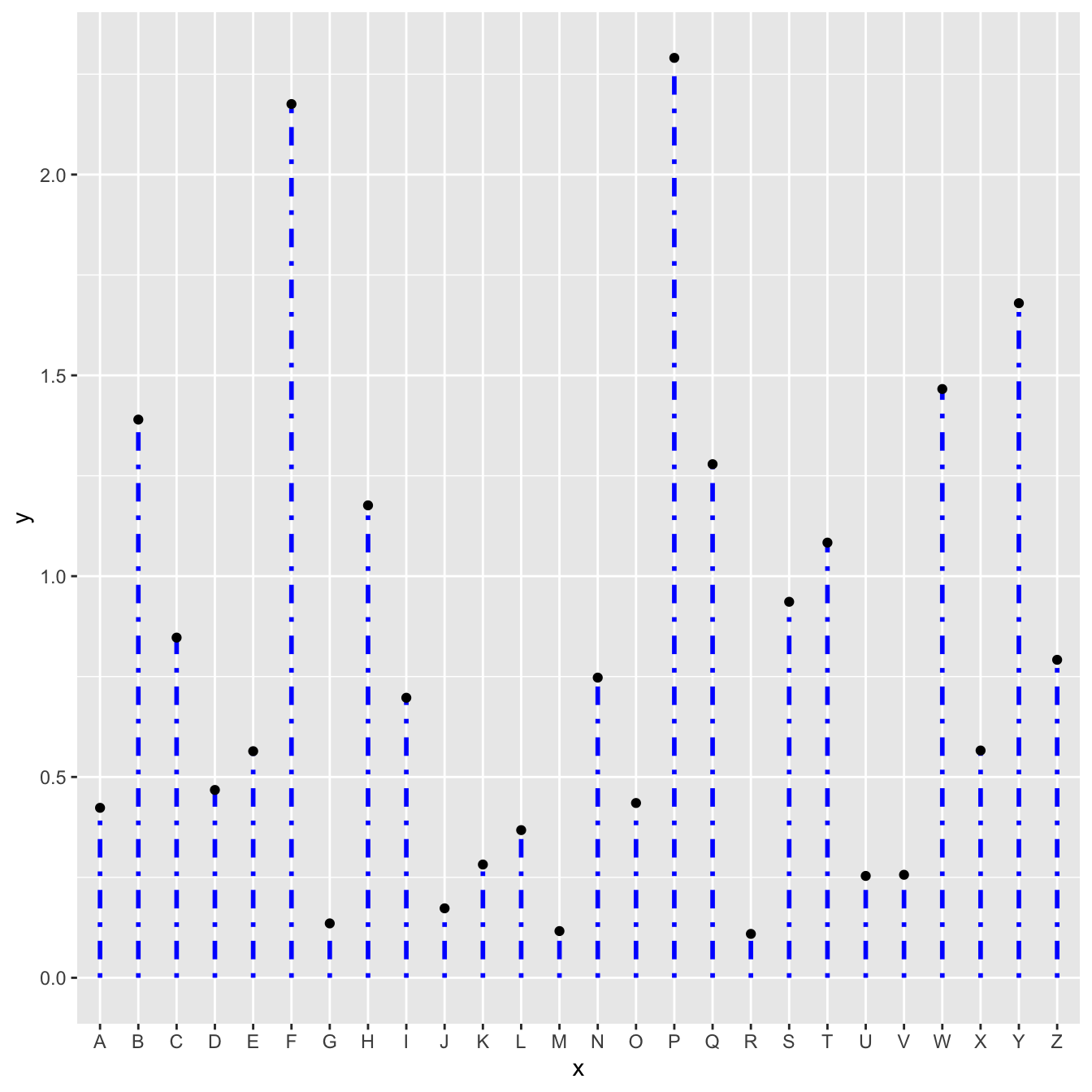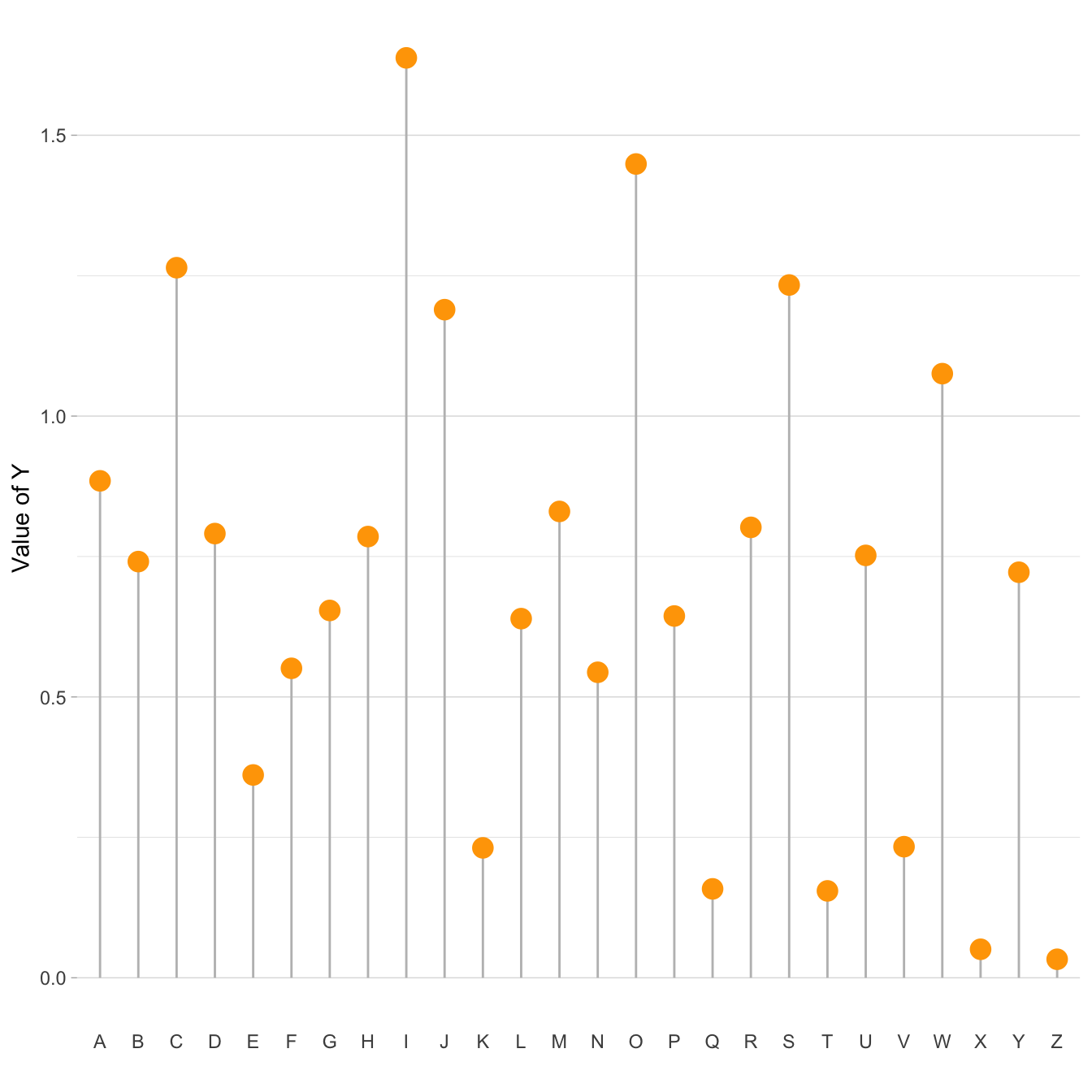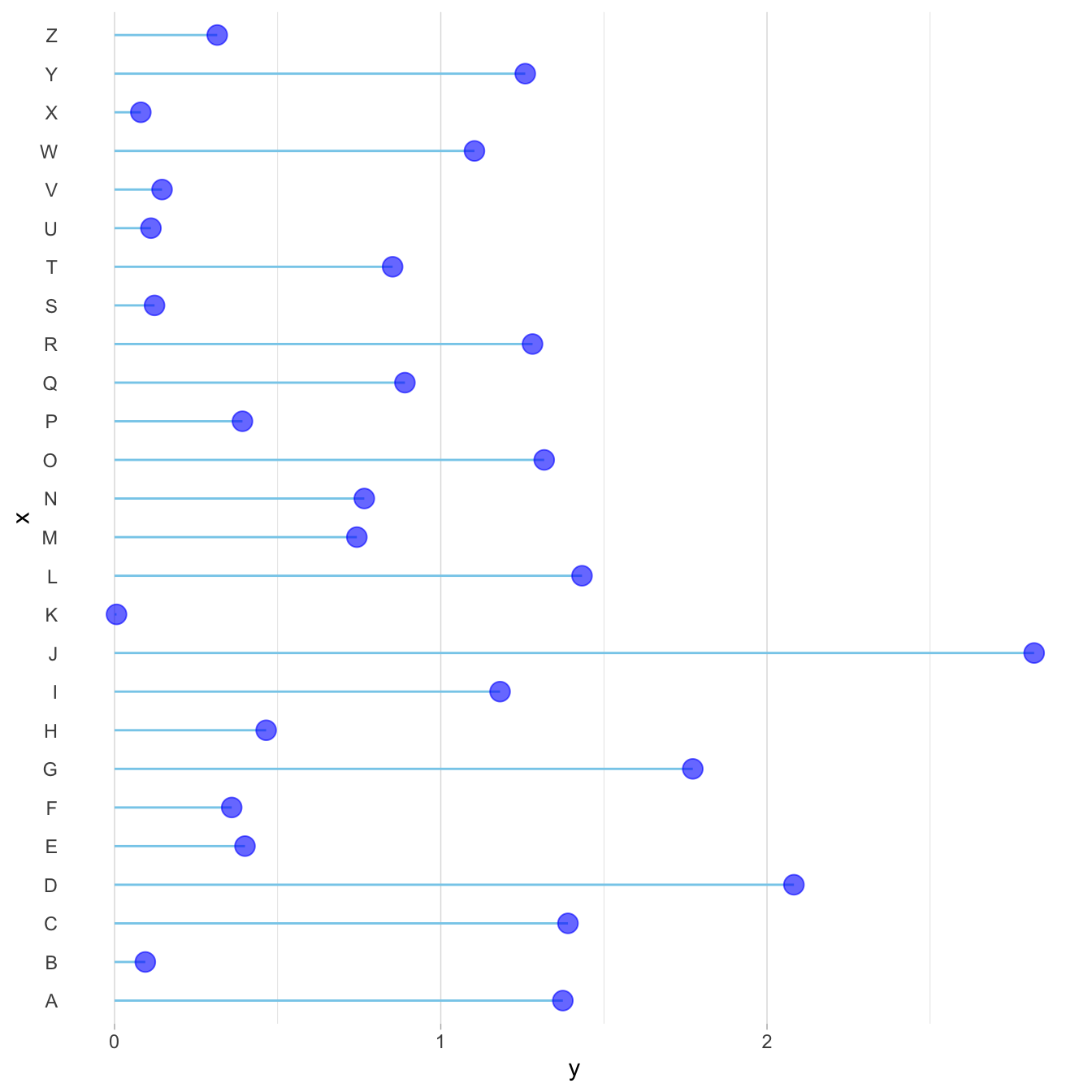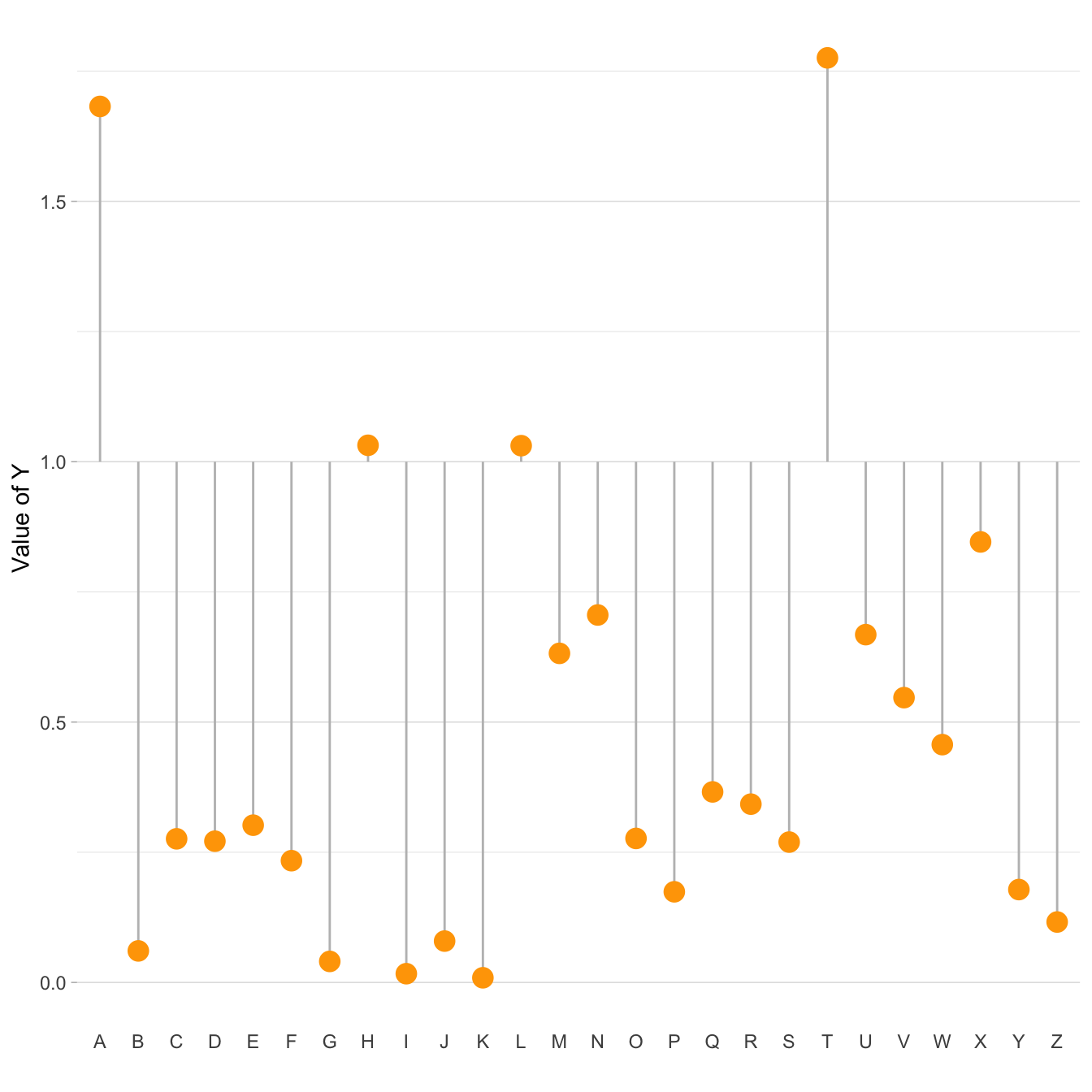# Custom lollipop chart

A lollipop chart is constituted of a circle (made with `geom_point()`) and a segment (made with `geom_segment()`). This page explains how to customize the chart appearance with `R` and `ggplot2`.

# Marker

A lollipop plot is constituted of a marker and a stem. You can customize the marker as usual with ggplot2:

• `size`, `color`
• `alpha` → transparency
• `shape` → see list of available shape here
• `stroke` and `fill` → only for shapes that have stroke, like the `21```````# Library
library(tidyverse)

# Create data
data <- data.frame(
x=LETTERS[1:26],
y=abs(rnorm(26))
)

# plot
ggplot(data, aes(x=x, y=y)) +
geom_segment( aes(x=x, xend=x, y=0, yend=y)) +
geom_point( size=5, color="red", fill=alpha("orange", 0.3), alpha=0.7, shape=21, stroke=2) ``````

# Stem

The stem is built using `geom_segment()` and can be customized as well:

• `size`, `color`
• `linetype` → can be an integer (see list), a word like `dotted`, `dashed`, `dotdash` and more (type `help(linetype)`)``````# Libraries
library(ggplot2)

# Create data
data <- data.frame(
x=LETTERS[1:26],
y=abs(rnorm(26))
)

# Plot
ggplot(data, aes(x=x, y=y)) +
geom_segment( aes(x=x, xend=x, y=0, yend=y) , size=1, color="blue", linetype="dotdash" ) +
geom_point()``````

# General appearance with `theme()`

As usual, you can customize the general appearance of the chart using the `theme()` function.

Note: another solution is to use the pre-built `theme_ipsum()` offered in the `hrbrthemes` package.``````# Libraries
library(ggplot2)

# Create data
data <- data.frame(
x=LETTERS[1:26],
y=abs(rnorm(26))
)

# Plot
ggplot(data, aes(x=x, y=y)) +
geom_segment( aes(x=x, xend=x, y=0, yend=y), color="grey") +
geom_point( color="orange", size=4) +
theme_light() +
theme(
panel.grid.major.x = element_blank(),
panel.border = element_blank(),
axis.ticks.x = element_blank()
) +
xlab("") +
ylab("Value of Y")``````

# Horizontal version

It is pretty straightforward to flip the chart using the `coord_flip()` function.

It makes sense to do so if you have long labels → they will be much easier to read.``````# Libraries
library(ggplot2)

# Create data
data <- data.frame(
x=LETTERS[1:26],
y=abs(rnorm(26))
)

# Horizontal version
ggplot(data, aes(x=x, y=y)) +
geom_segment( aes(x=x, xend=x, y=0, yend=y), color="skyblue") +
geom_point( color="blue", size=4, alpha=0.6) +
theme_light() +
coord_flip() +
theme(
panel.grid.major.y = element_blank(),
panel.border = element_blank(),
axis.ticks.y = element_blank()
)``````

# Baseline

Lastly, you can easily change the baseline of the chart. It gives more insight to the figure if there is a specific threshold in the data that interests you.

You just have to change the `y` argument in the `geom_segment()` call.``````# Libraries
library(ggplot2)

# Create data
data <- data.frame(
x=LETTERS[1:26],
y=abs(rnorm(26))
)

# Change baseline
ggplot(data, aes(x=x, y=y)) +
geom_segment( aes(x=x, xend=x, y=1, yend=y), color="grey") +
geom_point( color="orange", size=4) +
theme_light() +
theme(
panel.grid.major.x = element_blank(),
panel.border = element_blank(),
axis.ticks.x = element_blank()
) +
xlab("") +
ylab("Value of Y")``````

# What’s next

The lollipop chart is one of my favourite. There is so much to do with it and it is under-utilized in favor of barplot. Visit the dedicated section for more examples produced with `R`, or data-to-viz to learn about the available variations and caveats to avoid.

Related chart types

## Contact

This document is a work by Yan Holtz. Any feedback is highly encouraged. You can fill an issue on Github, drop me a message on Twitter, or send an email pasting yan.holtz.data with gmail.com.